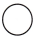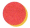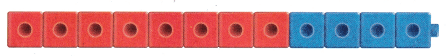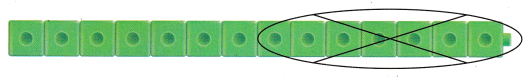Refer to our Texas Go Math Grade 1 Answer Key Pdf to score good marks in the exams. Test yourself by practicing the problems from Texas Go Math Grade 1 Module 8 Assessment Answer Key.

Concepts and Skills

There are 15 children eating lunch.
____ children do not buy lunch.
Explanation:
7 children do not buy lunch.

Question 1.
Choose numbers to generate an addition story problem. Write the addition sentence to match the story problem. TEKS 1.3.F ________ = 15
15 children went to a picnic.8 bought lunch and 7 did not bought lunch
7 + 8 = 15
Explanation:
Written a story problem

Question 2.
Choose numbers to generate a subtraction story problem. Write the subtraction sentence to match the story problem. TEKS 1.3.F
15___ = ____
15 children went to a picnic. Some bought lunch and 7 did not bought lunch
15 – 7 = 8
Explanation:
Written a story problem

Question 3.
Useor objects to model the problem. Draw theor objects. Kaelin has 9 stickers. She gets 2 more stickers. Then Kaelin gives away 4 stickers. How many stickers does she have now? Explain the strategy you used to
solve the problem. TEKS 1.3.E
____ stickers
9 + 2 = 11
11 – 4 = 7
Explanation:
She has now 7 stickers.

Texas Test Prep

Question 4.
Which addition sentence matches the model? TEKS 1.3.F(A) 4 + 3 = 7
(B) 8 + 4 = 12
(C) 9 + 2 = 11
Explanation:
8 + 4 = 12

Question 5.
Which subtraction sentence matches the model? TEKS 1.3.F(A) 12 – 7 = 5
(B) 18 – 9 = 9
(C) 14 – 7 = 7
Explanation:
14 – 7 = 7
matches the subtraction sentence.

Question 6.
Michaela has 6 birds in the cage. There are 13 birds in the cage. How many birds do not belong to Michaela? TEKS 1.3.E
(A) 13 – 6 = 7
(B) 9 + 4 = 13
(C) 13 – 5 = 8